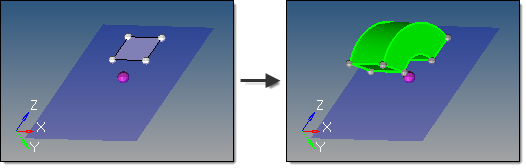﻿ spin subpanel (Solids panel)
Manufacturing Solutions

# spin subpanel (Solids panel)# spin subpanel (Solids panel)This subpanel creates solids by spinning surfaces around an axis.This example spins the selected surface 120 degrees around the X axis at the base node, in the positive direction.

Seven inputs are required to create a solid using this method:

 • The surfaces to spin.

No solid faces may be selected as input.

 • The merge solids at shared edges option applies when one or more input surfaces are attached to each other.  This does not apply to single surface or unconnected surface selections.

If disabled, a solid is created for each input surface, with shared faces created at the shared edge locations.

If enabled, a single solid is created with merged faces created at the shared edge locations.

 • The create in method, which defines the resulting solids component organization.

Specifying current component organizes the new solids and the selected surfaces to the current component.

Specifying surfs component adds the new solids to the same component that the selected surfaces already belong to.  The result is unpredictable if surfaces from different components become a part of the same solid.

 • The plane/vector defining the rotation axis.  If a vector is defined or selected, this represents the axis of rotation.  If a plane is defined, the plane normal represents the axis of rotation.

The base node of the plane/vector represents the center of rotation.

 • The start angle defines the initial angle of the solid formed by the spin, measured about the axis of rotation using the right-hand rule. The selected surfaces will be rotated this many degrees before Altair HyperMesh extrudes the solid from them by an additional number of degrees determined by the difference between the start angle and end angle. Thus, each angle determines the position of one face along the arc of rotation, and therefore the difference between start and end angles determines the thickness of the solid through the arc.
 • The end angle defines the final angle through with the surfaces are spun, thus determining the solid's thickness along the arc of rotation.  The angle is measured about the axis of rotation using the right-hand rule.  The total angle is given by (end angle - start angle).
 • The direction of the spin.

Spin + is defined using the right-hand rule around the axis of rotation and uses the start angle and end angle values as specified.

Spin - is defined in the opposite direction and uses the negative of the specified start angle and end angle values.